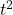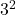## 3. Daniel stands on a bridge over a river. He drops a coin and notes that the coin takes 3 s to reach the river below. He must b

Question

3. Daniel stands on a bridge over a river. He drops a coin and notes that the coin takes 3 s to reach the river below.

He must be standing

above the river.

A 10 m

B. 15 m

C. 30 m

D. 45 m

in progress 0
2 months 2021-07-30T03:08:10+00:00 1 Answers 4 views 0

45 m

Explanation:

Initial velocity of the coin = 0 m/s  (since he dropped it from his hands)

time spent in falling – 3 sec

distance s = ?

using

s = ut +gwhere

s is the distance the stone fell

u is the initial velocity

t is the time spent falling

g is acceleration due to gravity = 10 m/s^2 (positive downwards)

substituting values, we have

s = (0 x 3) +(10 x)

s = 45 m